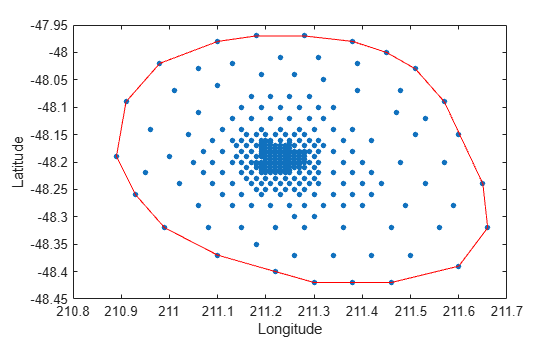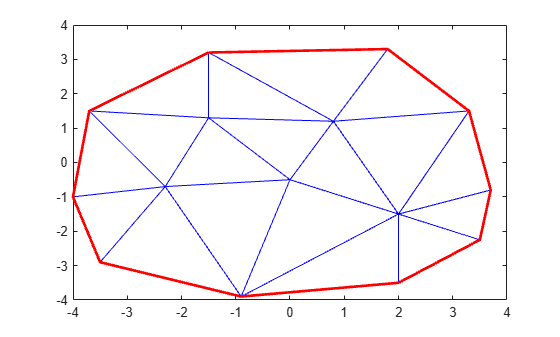Computing the Convex Hull

MATLAB® provides several ways to compute the convex hull:

The convhull function supports the computation of convex hulls in 2-D and 3-D. The convhulln function supports the computation of convex hulls in N-D (N ≥ 2). The convhull function is recommended for 2-D or 3-D computations due to better robustness and performance.

The delaunayTriangulation class supports 2-D or 3-D computation of the convex hull from the Delaunay triangulation. This computation is not as efficient as the dedicated convhull and convhulln functions. However, if you have a delaunayTriangulation of a point set and require the convex hull, the convexHull method can compute the convex hull more efficiently from the existing triangulation.

The alphaShape function also supports the 2-D or 3-D computation of the convex hull by setting the alpha radius input parameter to Inf. Like delaunayTriangulation, however, computing the convex hull using alphaShape is less efficient than using convhull or convhulln directly. The exception is when you are working with a previously created alpha shape object.

Computing the Convex Hull Using convhull and convhulln

The convhull and convhulln functions take a set of points and output the indices of the points that lie on the boundary of the convex hull. The point index-based representation of the convex hull supports plotting and convenient data access. The following examples illustrate the computation and representation of the convex hull.

The first example uses a 2-D point set from the seamount dataset as input to the convhull function.

Compute the convex hull of the point set.

K = convhull(x,y);

K represents the indices of the points arranged in a counter-clockwise cycle around the convex hull.

Plot the data and its convex hull.

plot(x,y,'.','markersize',12)
xlabel('Longitude')
ylabel('Latitude')
hold on

plot(x(K),y(K),'r')Add point labels to the points on the convex hull to observe the structure of K.

[K,A] = convhull(x,y);

convhull can compute the convex hull of both 2-D and 3-D point sets. You can reuse the seamount dataset to illustrate the computation of the 3-D convex hull.

Include the seamount z-coordinate data elevations.

close(gcf)
K = convhull(x,y,z);

In 3-D the boundary of the convex hull, K, is represented by a triangulation. This is a set of triangular facets in matrix format that is indexed with respect to the point array. Each row of the matrix K represents a triangle.

Since the boundary of the convex hull is represented as a triangulation, you can use the triangulation plotting function trisurf.

trisurf(K,x,y,z,'Facecolor','cyan')The volume bounded by the 3-D convex hull can optionally be returned by convhull, the syntax is as follows.

[K,V] = convhull(x,y,z);

The convhull function also provides the option of simplifying the representation of the convex hull by removing vertices that do not contribute to the area or volume. For example, if boundary facets of the convex hull are collinear or coplanar, you can merge them to give a more concise representation. The following example illustrates use of this option.

[x,y,z] = meshgrid(-2:1:2,-2:1:2,-2:1:2);
x = x(:);
y = y(:);
z = z(:);

K1 = convhull(x,y,z);
subplot(1,2,1)
defaultFaceColor  = [0.6875 0.8750 0.8984];
trisurf(K1,x,y,z,'Facecolor',defaultFaceColor)
axis equal
title(sprintf('Convex hull with simplify\nset to false'))

K2 = convhull(x,y,z,'simplify',true);
subplot(1,2,2)
trisurf(K2,x,y,z,'Facecolor',defaultFaceColor)
axis equal
title(sprintf('Convex hull with simplify\nset to true'))MATLAB provides the convhulln function to support the computation of convex hulls and hypervolumes in higher dimensions. Though convhulln supports N-D, problems in more than 10 dimensions present challenges due to the rapidly growing memory requirements.

The convhull function is superior to convhulln in 2-D and 3-D as it is more robust and gives better performance.

Convex Hull Computation Using the delaunayTriangulation Class

This example shows the relationship between a Delaunay triangulation of a set of points in 2-D and the convex hull of that set of points.

The delaunayTriangulation class supports computation of Delaunay triangulations in 2-D and 3-D space. This class also provides a convexHull method to derive the convex hull from the triangulation.

Create a Delaunay triangulation of a set of points in 2-D.

X = [-1.5 3.2; 1.8 3.3; -3.7 1.5; -1.5 1.3; 0.8 1.2; ...
3.3 1.5; -4.0 -1.0; -2.3 -0.7; 0 -0.5; 2.0 -1.5; ...
3.7 -0.8; -3.5 -2.9; -0.9 -3.9; 2.0 -3.5; 3.5 -2.25];

dt = delaunayTriangulation(X);

Plot the triangulation and highlight the edges that are shared only by a single triangle reveals the convex hull.

triplot(dt)

fe = freeBoundary(dt)';
hold on
plot(X(fe,1), X(fe,2), '-r', 'LineWidth',2)
hold offIn 3-D, the facets of the triangulation that are shared only by one tetrahedron represent the boundary of the convex hull.

The dedicated convhull function is generally more efficient than a computation based on the convexHull method. However, the triangulation based approach is appropriate if:

• You have a delaunayTriangulation of the point set already and the convex hull is also required.

• You need to add or remove points from the set incrementally and need to recompute the convex hull frequently after you have edited the points.

Convex Hull Computation Using alphaShape

This example shows how to compute the convex hull of a 2-D point set using the alphaShape function.

alphaShape computes a regularized alpha shape from a set of 2-D or 3-D points. You can specify the alpha radius, which determines how tightly or loosely the alpha shape envelops the point set. When the alpha radius is set to Inf, the resulting alpha shape is the convex hull of the point set.

Create a set of 2-D points.

X = [-1.5 3.2; 1.8 3.3; -3.7 1.5; -1.5 1.3; 0.8 1.2; ...
3.3 1.5; -4.0 -1.0; -2.3 -0.7; 0 -0.5; 2.0 -1.5; ...
3.7 -0.8; -3.5 -2.9; -0.9 -3.9; 2.0 -3.5; 3.5 -2.25];

Compute and plot the convex hull of the point set using an alpha shape with alpha radius equal to Inf.

shp = alphaShape(X,Inf);
plot(shp)